# How to Calculate and Solve for the Centroid or Centre of Gravity of a Sphere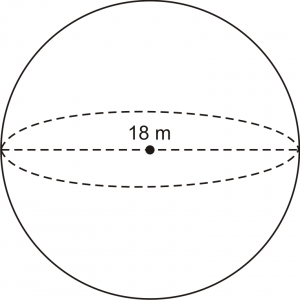The image above is a sphere and 18 m is the diameter of the sphere. In the computing of the centroid or centre of gravity of a sphere there is only one essential parameter which is the diameter of the sphere.

The formula for calculating the centroid or centre of gravity of a sphere is:

C.G. = d / 2

Where:

d is the diameter of the sphere

As always, let’s take an example:

Let’s solve an example
Find the centroid or centre of gravity of a sphere where the diameter is 18 m.

C.G. = 18 / 2
C.G. = 9

Therefore, the centroid or centre of gravity of the sphere is 9.

Nickzom Calculator – The Calculator Encyclopedia is capable of calculating the centre of gravity of a sphere at a distance from every point.

To get the answer and workings of the center of gravity or centroid of a sphere using the Nickzom Calculator – The Calculator Encyclopedia. First, you need to obtain the app.

You can get this app via any of these means:

To get access to the professional version via web, you need to register and subscribe for NGN 1,500 per annum to have utter access to all functionalities.
You can also try the demo version via https://www.nickzom.org/calculator

Once, you have obtained the calculator encyclopedia app, proceed to the Calculator Map, then click on Mechanics under the Engineering section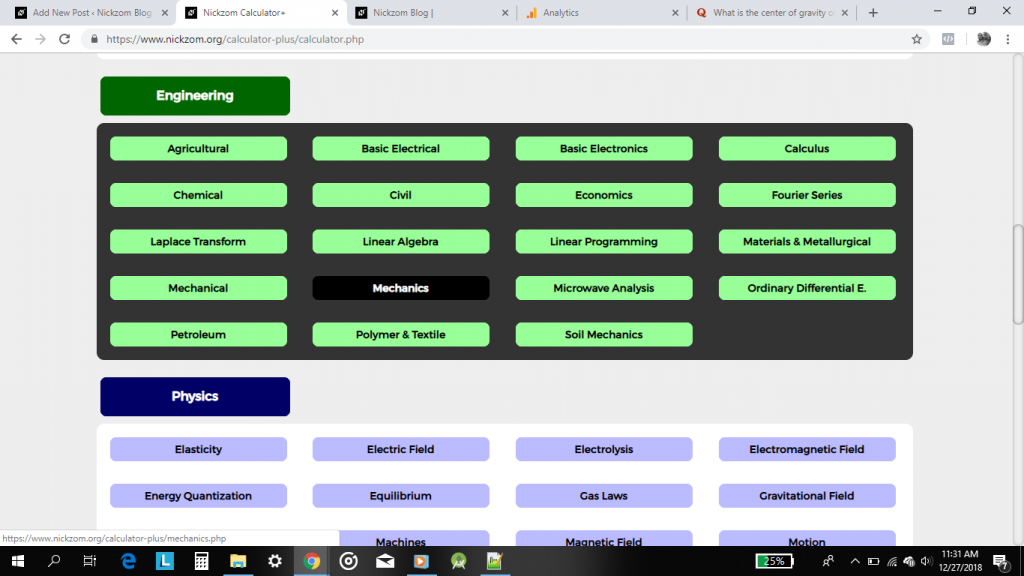Now, click on Centre of Gravity under Statics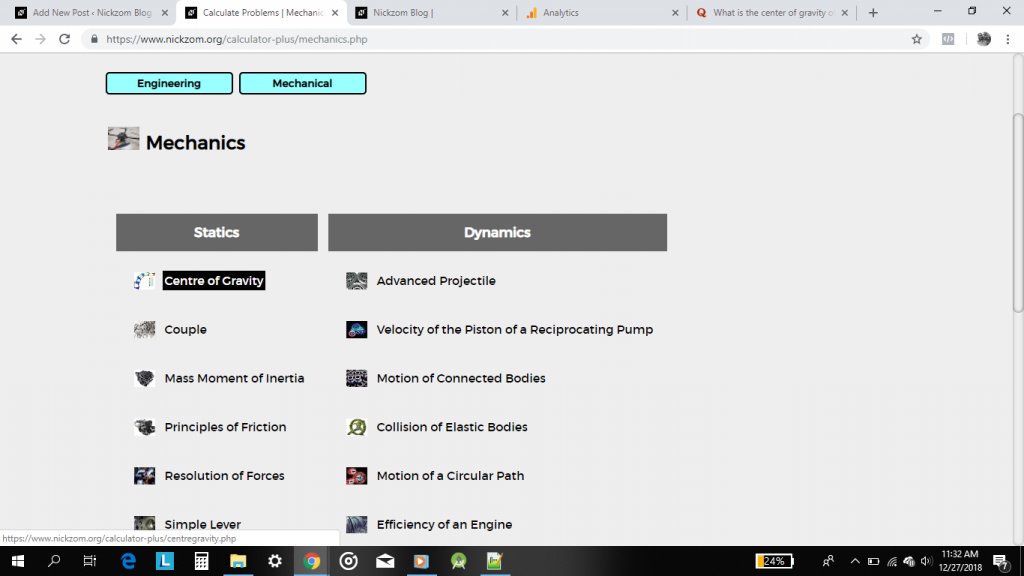Now, click on Centre of Gravity of a Sphere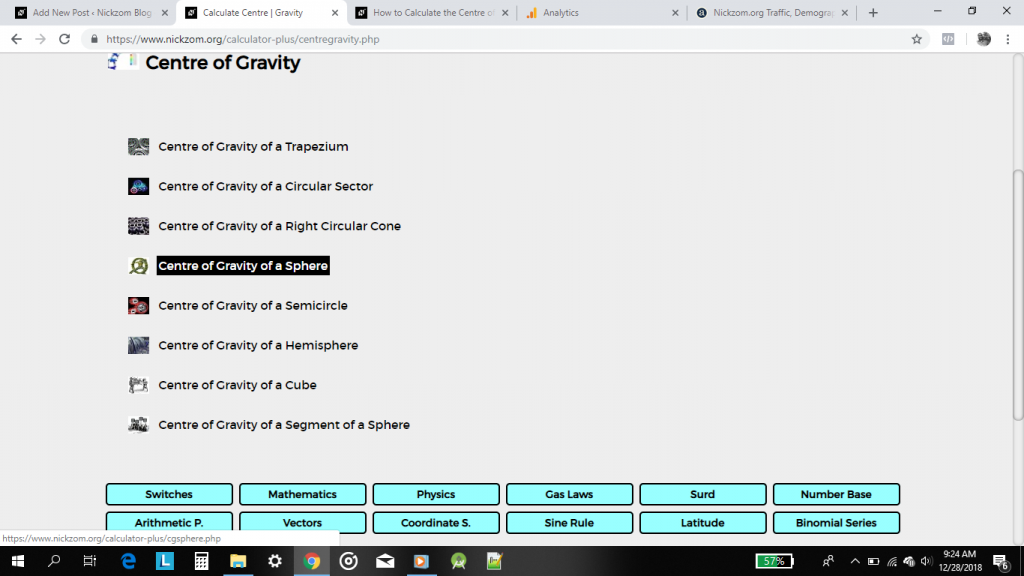The screenshot below displays the page or activity to enter your value to get answer for centre of gravity of a sphere according to the respective parameter which is the diameter of the sphere (d).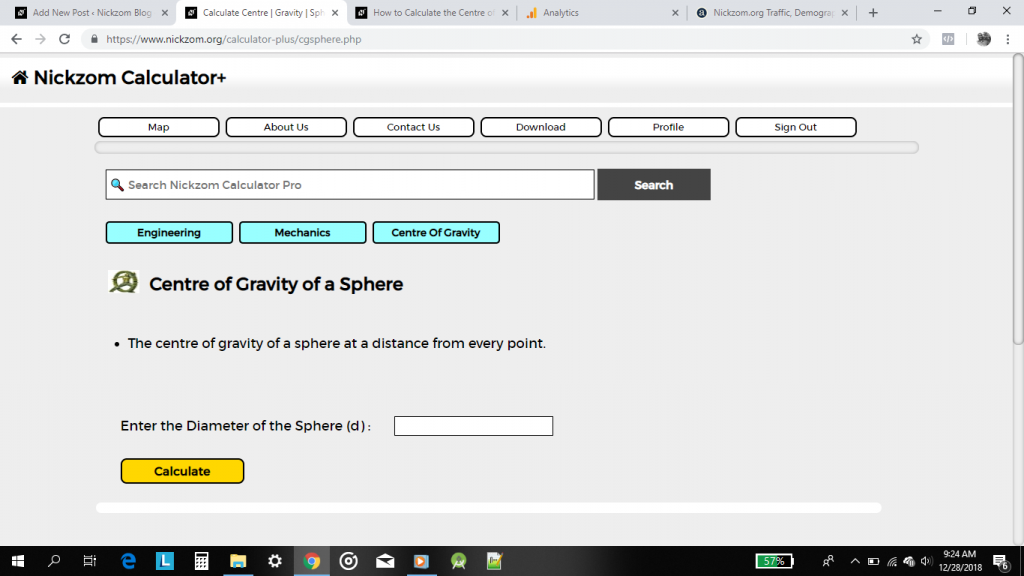Now, enter the value appropriately and accordingly for the parameter as required by the example above where the diameter of the sphere is 18.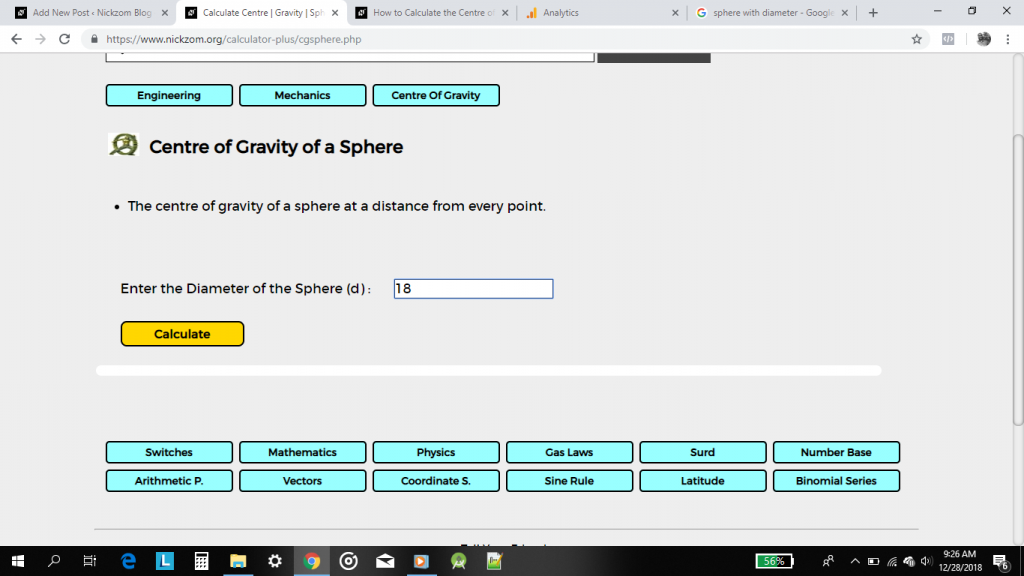Lastly, click on the Calculate button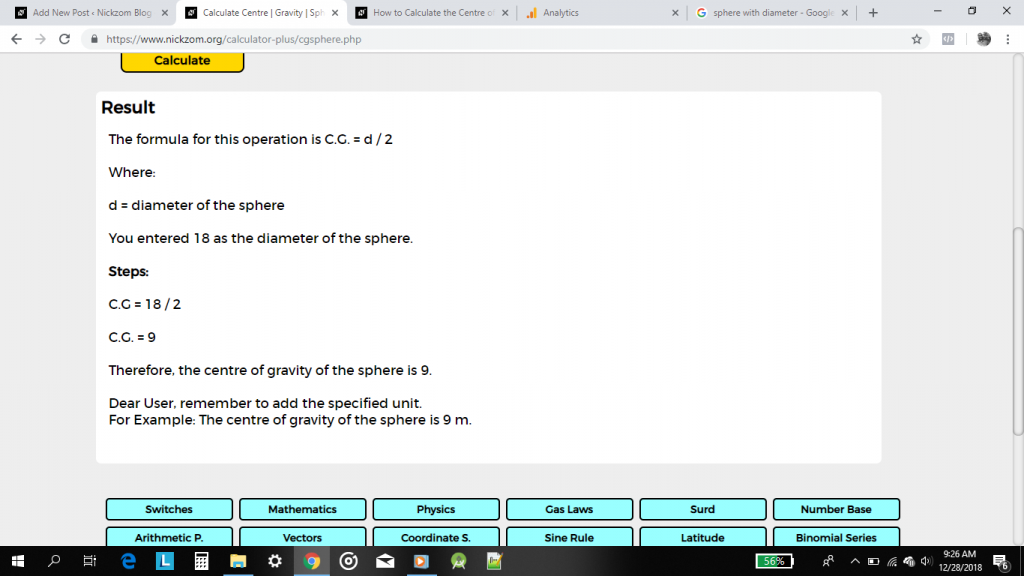As you can see from the screenshot above, Nickzom Calculator – The Calculator Encyclopedia solves for the centre of gravity or centroid of a sphere and presents the formula, workings and steps too.